##### Economic Indicators For DummiesAfter determining cash flows and the cost of capital, managers can begin to evaluate various capital investment alternatives. The most commonly employed technique for evaluating investment alternatives is the net present value technique. Variations of this technique include the profitability index and the internal rate of return.

## How to determine today’s net present value

The net present value (NPV) technique is based on the concept that you prefer receiving cash today rather than in the future. One obvious reason for this preference is the fact that cash received today can earn interest through the purchase of government securities or other types of bonds. Therefore, any future cash you receive needs to be discounted by an appropriate interest rate.

If all the capital investment project’s expenditures occur during the current year, the project’s net present value (NPV) equals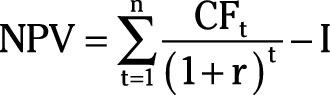where CFt represents the net after-tax cash flow in year t, r is the cost of capital, and I is the capital investment project’s cash outlay assumed to occur in the current year, or year 0.

If the capital investment project has any salvage value at the end of its use, the salvage value is included in the last year’s cash flow.

Any investment project with a positive net present value is profitable for the firm and should be undertaken.

For example, you’re evaluating a capital investment project that generates cash flows the next four years. Net cash flow estimates are \$18,000 the first year, \$45,000 the second year, \$50,000 the third year, and \$12,000 the fourth and final year. The capital project’s initial investment is \$105,000 and there is no salvage value at the end. Finally, the cost of capital is 8 percent.

In order to determine whether or not to undertake the project, you take the following steps:

1. Determine the present value for the first year’s cash flow.

Divide the first year’s net cash flow by (1 + r)1.2. Determine the present values for the second, third, and fourth years’ cash flow.

Note how the exponent changes in the denominator of the calculation.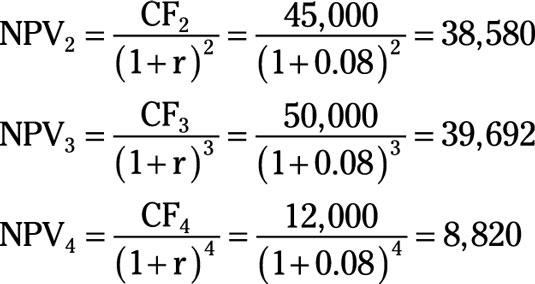3. Add the four years’ present values.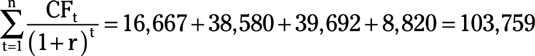4. Determine the capital investment project’s net present value.

Subtract the initial investment required from the sum of the four years’ present values.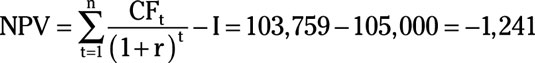5. Don’t make the capital investment.

Because the project’s net present value is negative, you shouldn’t invest in the project.

You must consider the time value of money when investing in a capital project. In this example, the sum of the undiscounted net cash flows is \$125,000, which is greater than the \$105,000 required investment. But remember that money received in the future isn’t worth as much as money received today.

As an alternative to the project, you could buy a government bond today and earn 8-percent interest or \$8,400 (0.08 × 105,000) annually.

## How to index profitability

The profitability index is a variation of the net present value technique. The profitability index (PI) is calculated as follows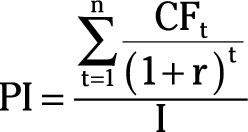where PI represents the profitability index, CFt represents the net after-tax cash flow in year t, r is the cost of capital, and I is the capital investment project’s cash outlay assumed to occur in the current year, or year 0.

Any project with a profitability index greater than 1 (PI>1)should be undertaken because the present value of its future cash flow exceeds the current investment — the numerator is larger than the denominator.

An important advantage of the profitability index is realized when circumstances prevent you from undertaking all profitable investments. In these situations, you use the profitability index to rank projects, undertaking projects with the highest profitability index first.

You’re evaluating a capital investment project that generates cash flows the next three years. Net cash flows are estimated to be \$1 million the first year, \$4 million the second year, and \$3 million the third and final year. The capital project’s initial investment is \$6 million and there is no salvage value at the end. Finally, the cost of capital is 10 percent.

In order to determine whether or not to undertake the project, you take the following steps:

1. Determine the present value for the firm’s future net cash flow.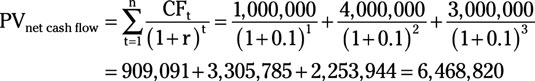2. Divide the project’s present value of future cash flows by the project’s cost.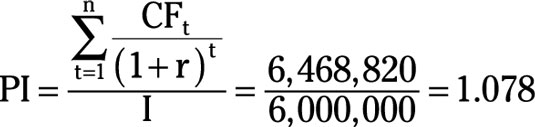3. Because the profitability index is greater than 1, you should undertake the project.

An index value greater than 1 indicates the present value of future cash flows exceeds the project’s initial cost.

One problem with the profitability index is that it tends to be biased in favor of smaller projects. For example, a project that requires a \$50,000 investment and has a present value of net cash flow equal to \$60,000 has a profitability index of 1.20.

On the other hand, a project requiring a \$5,000,000 investment that has a present value of net cash flow equal to \$5,500,000 has a profitability index of only 1.10. Clearly, the \$500,000 additional profit from the second project is better than the \$10,000 additional profit from the first project even though the second project has a lower profitability index.

## How to calculate the internal rate of return

Calculating the internal rate of return is another alternative application of the net present value technique. The internal rate of return is the interest rate that equates the project’s present value of future net cash flows with the project’s initial investment outlay. Mathematically,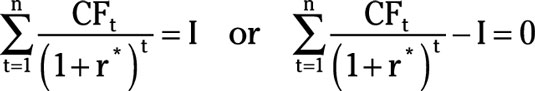The equation is solved for r*, which represents the internal rate of return.

If the project’s internal rate of return exceeds the cost of capital, the firm should undertake the project. This is similar to the situation described earlier.

The solution for the internal rate of return when done by hand is typically a laborious trial-and-error process. However, computers can be used to quickly solve this type of problem.